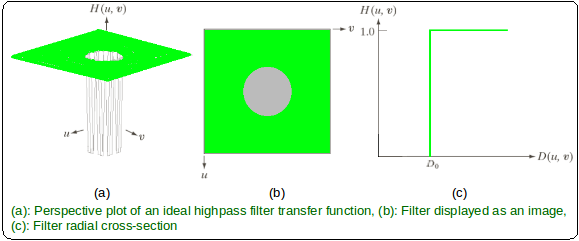# MATLAB – Ideal Highpass Filter in Image Processing

In the field of Image Processing, Ideal Highpass Filter (IHPF) is used for image sharpening in the frequency domain. Image Sharpening is a technique to enhance the fine details and highlight the edges in a digital image. It removes low-frequency components from an image and preserves high-frequency components.

This ideal highpass filter is the reverse operation of the ideal lowpass filter. It can be determined using the following relation-where,is the transfer function of the highpass filter andis the transfer function of the corresponding lowpass filter.

The transfer function of the IHPF can be specified by the function-Where,

•is a positive constant. IHPF passes all the frequencies outside of a circle of radiusfrom the origin without attenuation and cuts off all the frequencies within the circle.
• Thisis the transition point between H(u, v) = 1 and H(u, v) = 0, so this is termed as cutoff frequency.
•is the Euclidean Distance from any point (u, v) to the origin of the frequency plane, i.e,Approach:

Step 1: Input – Read an image
Step 2: Saving the size of the input image in pixels
Step 3: Get the Fourier Transform of the input_image
Step 4: Assign the Cut-off FrequencyStep 5: Designing filter: Ideal High Pass Filter
Step 6: Convolution between the Fourier Transformed input image and the filtering mask
Step 7: Take Inverse Fourier Transform of the convoluted image
Step 8: Display the resultant image as output

Implementation in MATLAB:

 % MATLAB Code | Ideal High Pass Filter     % Reading input image : input_image   input_image = imread('[name of input image file].[file format]');     % Saving the size of the input_image in pixels-  % M : no of rows (height of the image)  % N : no of columns (width of the image)  [M, N] = size(input_image);     % Getting Fourier Transform of the input_image  % using MATLAB library function fft2 (2D fast fourier transform)    FT_img = fft2(double(input_image));     % Assign Cut-off Frequency    D0 = 10; % one can change this value accordingly     % Designing filter  u = 0:(M-1);  idx = find(u>M/2);  u(idx) = u(idx)-M;  v = 0:(N-1);  idy = find(v>N/2);  v(idy) = v(idy)-N;     % MATLAB library function meshgrid(v, u) returns 2D grid  %  which contains the coordinates of vectors v and u.   % Matrix V with each row is a copy of v, and matrix U   % with each column is a copy of u  [V, U] = meshgrid(v, u);     % Calculating Euclidean Distance  D = sqrt(U.^2+V.^2);     % Comparing with the cut-off frequency and   % determining the filtering mask  H = double(D > D0);     % Convolution between the Fourier Transformed image and the mask  G = H.*FT_img;     % Getting the resultant image by Inverse Fourier Transform  % of the convoluted image using MATLAB library function  % ifft2 (2D inverse fast fourier transform)    output_image = real(ifft2(double(G)));     % Displaying Input Image and Output Image  subplot(2, 1, 1), imshow(input_image),  subplot(2, 1, 2), imshow(output_image, [ ]);

Input Image –Output:My Personal Notes arrow_drop_upCheck out this Author's contributed articles.

If you like GeeksforGeeks and would like to contribute, you can also write an article using contribute.geeksforgeeks.org or mail your article to contribute@geeksforgeeks.org. See your article appearing on the GeeksforGeeks main page and help other Geeks.

Please Improve this article if you find anything incorrect by clicking on the "Improve Article" button below.

Article Tags :

Be the First to upvote.

Please write to us at contribute@geeksforgeeks.org to report any issue with the above content.